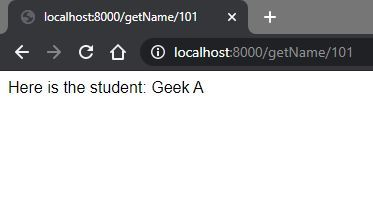Open In App

# How to convert an existing callback to a promise in Node.js ?

Callback: In terms of JavaScript, callback is a function passed as a parameter to another function. The function executes as soon as the result of the calling function gets ready. In simple words, it handles the asynchronous requirement of JavaScript.

Example: The best example to demonstrate this is to use a setTimeout() function which accepts a callback and delays the execution of JavaScript code. As soon as the time set to function gets over, the callback function executes.

 `// Defining a callback function ``var` `callback = ()=>{``    ``console.log(``"Hello! GeeksforGeeks"``);``}`` ` `// Passing the callback function``// as a parameter``setTimeout(callback, 2000);`` ` `// The callback gets executed as``// soon as 2 seconds are over.``// This behavior shows the ``// execution of a callback.`

Output:

`Hello! GeeksforGeeks`

Promises: It is very much similar to the callbacks in operation. But, the advantage of using Promises is to improve the code readability as it saves us from the callback hell.
Promises have four states

• Pending: The promise has not yet completed. It did not succeed and fail.
• Fulfilled: The promise ended with success.
• Rejected: The promise ended with an error.
• Settled: The promise either gave an error or succeeded.

Converting an existing callback to a Promise:

 `// Existing Callback``var` `callback = ``function``(err, success) {``    ``if``(err) {``        ``console.log(``"Geek is very sad!"``);``    ``}``    ``else` `{``        ``console.log(``"Geek is optimistic, "``            ``+ ``"thus becomes successful"``);``    ``}``}``  ` `var` `caller = ``function``(status, callback){``    ``if``(status === ``'Happy'``)``        ``callback(``null``, ``true``);``    ``else` `{``        ``callback(``new` `Error(), ``false``);``    ``} ``}``  ` `// Calling the caller method`` ` `// Executes the success part``caller(``'Happy'``, callback);`` ` `// Executes the error part``caller(``'Sad'``, callback);`

Output:

```Geek is optimistic, thus becomes successful

Steps to follow:

• Define a function named error and insert the error codeblock of callback function into it.
• Define a function named success and insert the success codeblock into it.
• Then modify the caller code by returning promise object.
• Use the success and error methods in any of the following ways.
• See the code snippet below for better understanding.

 `// This snippet briefly shows``// the implementation``var` `error = ``function``(){``     ` `    ``// The error codeblock from``    ``// the existing callback.``    ``console.log(``"Geek is very sad!"``);``}``    ` `var` `success = ``function``(){``     ` `    ``// The success codeblock from``    ``// the existing callback``    ``console.log(``"Geek is optimistic, "``        ``+ ``"thus becomes successful"``);``}``    ` `var` `caller = ``function``(status) {``    ``return` `new` `Promise(``function``(resolve, reject) {``        ``if``(status === ``'Happy'``) {``             ` `            ``// Calling the resolve function ``            ``// when function returns success``          ``resolve();``        ``}``        ``else` `{``          ` `            ``// Calling the reject function``            ``// when function returns failure``            ``reject();``        ``}``    ``});``};`` ` `// Throw success``caller(``'Happy'``).then(success).``catch``(error);`` ` `// Throw error``caller(``'Sad'``).then(success).``catch``(error);`

Output:

```Geek is optimistic, thus becomes successful

Let us change the following code snippet into a promise implementation. To make the implementation run, we need to set up a web app running on a node server.

To understand using an example we need to set up a node server on the system. You can follow the following steps to set up a node server.

Install node and set up a simple node application by following the steps as shown here.

Example: Here, the scenario is that we have a student array having id and name as values. We need to get the name of the student having the given id. We are given an existing callback that needs to be converted to a promise.

 `/* The following code snippet depicts a GET request``to the server made by the client, which requests for``the name of the student with a given student id. Here we``have a student array that stores JSON objects of``student id and name. We are going to create a GET``Request using expressjs in node first. Then we will``write a route to get name of student based on id. */``const express = require(``'express'``);``const app = express();`` ` `// Students array``var` `students = [``    ``{``        ``id: 101,``        ``name: ``"Geek A"``    ``},``    ``{``        ``id: 102,``        ``name: ``"Geek B"``    ``},``    ``{``        ``id: 103,``        ``name: ``"Geek C"``    ``},``    ``{``        ``id: 104,``        ``name: ``"Geek D"``    ``}``];`` ` `// Definition of the callback function``const callback = (err, student) => {``    ``if``(err) {``        ``return` ``Student ``with` `given id \${err} not found`;``    ``}``    ``else` `{``        ``return` `"Here is the student: "` `+ student.name;``    ``}``}`` ` `// Passing studentid and callback function as parameter``const findName = (studentId, callbackFunction) => {``    ``let student = students.find(``function``(studentValue) {``        ``return` `studentValue.id == studentId;``    ``});`` ` `    ``// Student not found``    ``if``(``typeof` `student === ``'undefined'``)  {``        ``return` `callbackFunction(studentId, ``false``);``    ``}``    ``else` `{  ``// Student found``       ``return` `callbackFunction(``null``, student);``    ``}``}``  ` `const getName = (req, res)=>{``     ` `    ``// Sending back the response to the server``    ``res.send(findName(req.params.studentId, callback));``}``  ` `app.get(``'/getName/:studentId'``, getName);``  ` `app.listen(8000, ``'localhost'``, ``function` `() {``    ``console.log(``'Server Listening'``);``});`

Output:Now, we can convert the callback function into promises. Here, we can follow the earlier steps again i.e. Error logic in the failure function and Success logic in the success function. Please see the below code for more clarity.

 `const express = require(``'express'``);``const app = express();``  ` `var` `students = [``    ``{``        ``id: 101,``        ``name: ``"Geek A"``    ``},``    ``{``        ``id: 102,``        ``name: ``"Geek B"``    ``},``    ``{``        ``id: 103,``        ``name: ``"Geek C"``    ``},``    ``{``        ``id: 104,``        ``name: ``"Geek D"``    ``}``];`` ` `// Writing the success logic here``const success = (student) => {``    ``return` `"Here is the student: "` `+ student.name;``}`` ` `// Writing the failure logic here.``const failure = (fail) => {``    ``return` ``Student ``with` `the given id \${fail} was not found`;``}``  ` `const findName = (studentId) => {``    ``return` `new` `Promise(``function` `(resolve, reject) {``        ``let student = students.find(``function` `(studentValue) {``            ``return` `studentValue.id == studentId;``        ``});``        ``if` `(student) {``            ``resolve(student);``        ``}``        ``else` `{``            ``reject(id);``        ``}``    ``});``}``  ` `const getName = async (req, res) => {``    ``let answer = await findName(``        ``req.params.studentId).then(success).``catch``(failure);``     ` `    ``res.send(answer);``}``  ` `app.get(``'/getName/:studentId'``, getName);``  ` `app.listen(8000, ``'localhost'``, ``function` `() {``    ``console.log(``'Server Listening'``);``});`

Output: